Next: Addition and Multiplication Up: Building Blocks Previous: Building Blocks

## Gaussian variables

A Gaussian variable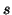has two inputs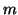and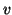and prior probability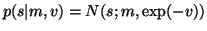. The variance is parametrised this way because then the mean and expected exponential ofsuffice for computing the cost function. It can be shown that when,andare mutually independent, i.e.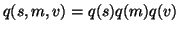,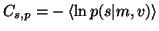yields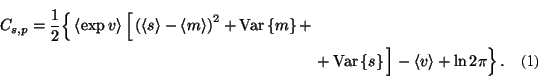For observed variables this is the only term in the cost function but for latent variables there is also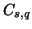: the part resulting from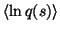. The posterior approximation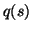is defined to be Gaussian with mean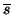and variance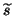: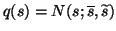. This yields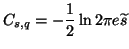(2)

which is the negative entropy of Gaussian variable with variance. The parametersandare to be optimised during learning.

The output of a latent Gaussian node trivially provides expectation and variance: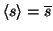and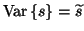. The expected exponential can be shown to be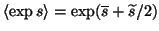. The outputs of observed nodes are scalar values instead of distributions and thus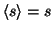,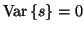and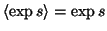.

The posterior distributionof a latent Gaussian node can be updated as follows. 1) First, the gradients of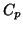w.r.t.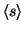,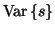and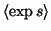are computed. 2) Second, the terms inwhich depend onandare assumed to be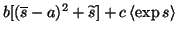, where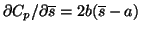,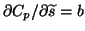and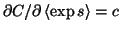. This assumption holds exactly if the output of the node is propagated to Gaussian nodes only and not to discrete nodes. If the output is used by a discrete node with a soft-max prior, this term gives an upper bound ofas will be explained later. 3) Third, the minimum of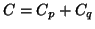is solved. This can be done analytically if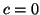, otherwise the minimum is obtained iteratively.Next: Addition and Multiplication Up: Building Blocks Previous: Building Blocks
Harri Valpola 2001-10-01